• CFD, Fluid Flow, FEA, Heat/Mass Transfer

Textbook Solutions: Fluid Mechanics, Heat & Mass Transfer, Aerodynamics

### FM, HTX, MTX, AERO, COMBUSTION

This page is being continuously updated with complex (text-book type) problems in Fluid Mechanics, Heat Transfer, Aerodynamics, Mass Transfer, Combustion and Thermodynamics. The solutions to compressible flows including sub-sonic, sonic and supersonic flows inside a converging-diverging nozzle will be presented.

#### Summary of Hydrostatics

• For a plane surface immersed in a liquid in any orientation, the total normal pressure over the surface is P = A * ρ * g * h where h is the vertical depth of the centre of the gravity of the surface from free surface of the liquid, A is area of the surface.
• A plane surface immersed in a liquid is acted upon by a system of parallel forces which can be reduced to act on a single point of the surface known as centre of pressure.
• A curved surface immersed in a liquid is not acted upon a "system of parallel forces" and hence in general they cannot be reduced to a single force.

#### Centre of pressure of vertical rectangular plate

This is a very useful case which has frequent engineering applications.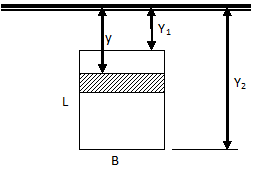From the definition of centre of pressure, the value can be calculated as: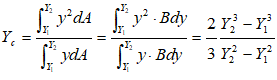Similarly, for a triangular plate with base horizontal and at depth of Y2 and vertex up at depth of Y1, the centre of pressure is given by: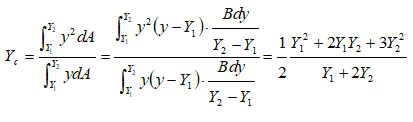Note that if the triangular plate is with base horizontal and at depth of Y1 and vertex down at depth of Y2, the centre of pressure can be obtained by interchanging Y1 and Y2 in above expression. Thus:#### Centre of pressure of vertical circular plate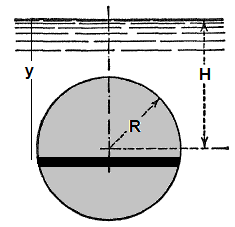Even though the complicated definite integration is possible analytically, this is the time the introduce a simple method based on properties of sections. Note that the numerator of expression for centre of pressure is the "Second Moment of Inertia" or simply the Moment of Inertia about a "chosen axis" - typically the free surface of the liquid. The denominator is the "Moment of Area" about the same axis as chosen above - also known as "Statical Moment". Thus:For the circular plate:Note about location of centre of pressure in the coordinate direction perpendicular to the depth of liquid column:
• If the area has axis of symmetry about the vertical axis, the centre of pressure will fall on it.
• Conversely, the centre of pressure will fall on the medial line, the vertical axis passing through the centre of gravity on the horizontal axis.
• The position of the centre of pressure is always below the centre of gravity of a surface.

#### Buoyancy - Example Problem

A metallic block is floating along with a wooden block as shown in the figure below. If this metallic block falls into the tank, calculate the new depth of floatation and height of water level in the tank.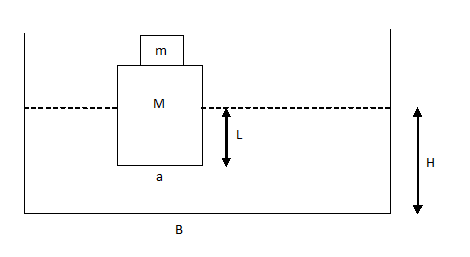Volume of liquid: VLIQ = A* H – a * L = constant. Total weight of wooden and metallic block = weight of displaced liquid. Thus: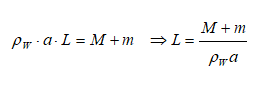Similarly, when upper block of mass m is removed:Now, change in H due to smaller block falling into the tank:#### Analogy Between Fluid Flow, HTX and MTX

The method of relating two phenomena with some come feature is a powerful tool to both understand and remember the concepts. Following table summarizes key parameters which are analogous in the field of fluid flow (momentum transfer), heat transfer and mass transfer.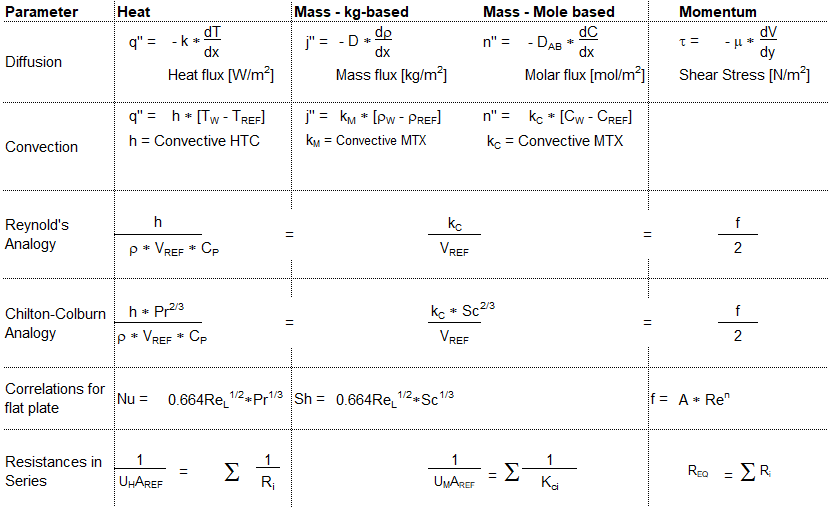#### 1D Heat Transfer with Convective Boundaries and Heat Generation

The designs of heat exchangers are the most predominant application of conductive heat transfer with such boundary conditions. The temperature profile in walls of a water-cooled internal combustion engine is also subject to this combination of boundary conditions though heat generation is not present in this application. Some applications can be current carrying conductor cooled by convection on both inner and outer diameters, nuclear fission in a annular cross-section cooled by convection on both the inner and outer radii.
The governing equation and general solution of the differential equation is given byHeat flow is assumed positive from left to right. Hence, the convective heat transfer on left face is negative if ambient temperature is < wall temperature on the left face!Finally, the temperature distribution function is expressed as: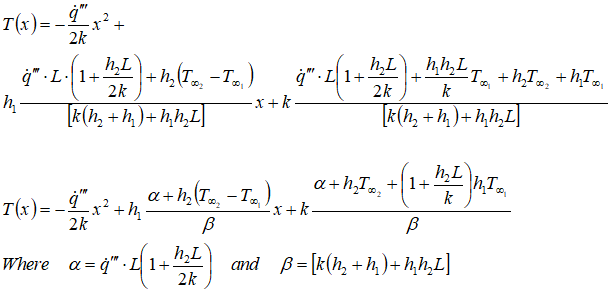#### Plug Flow between Parallel Plates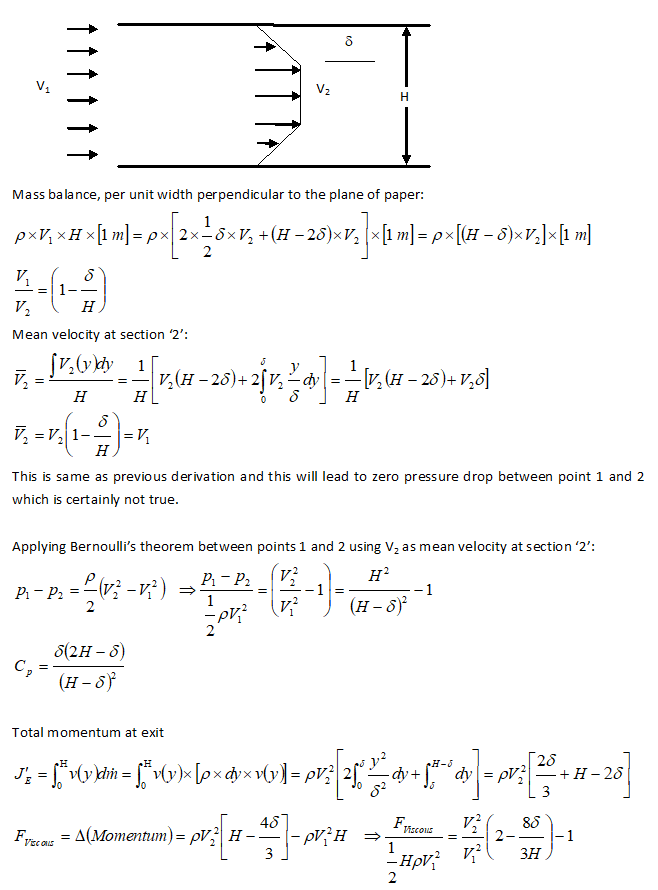#### Flow in an Annular Passage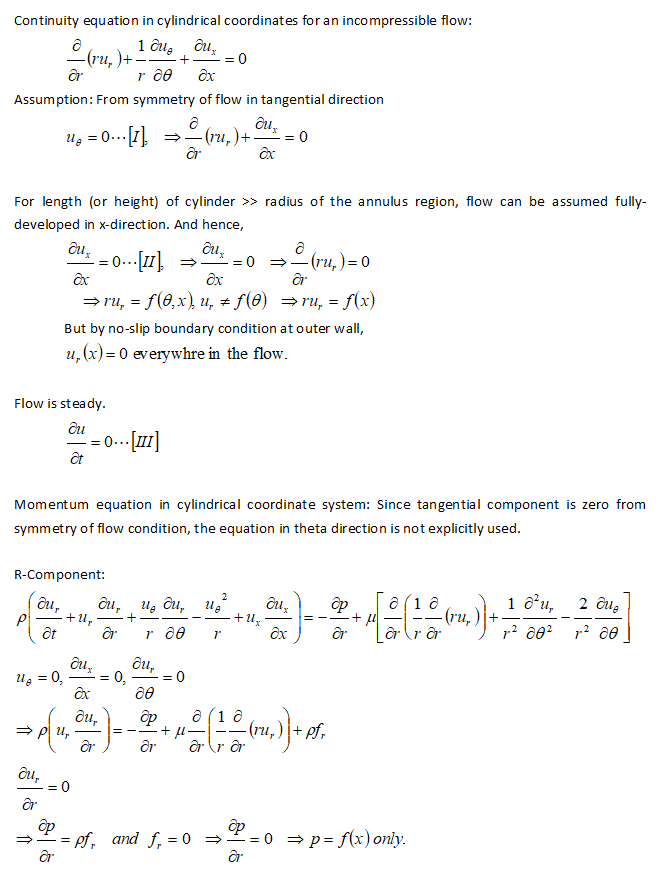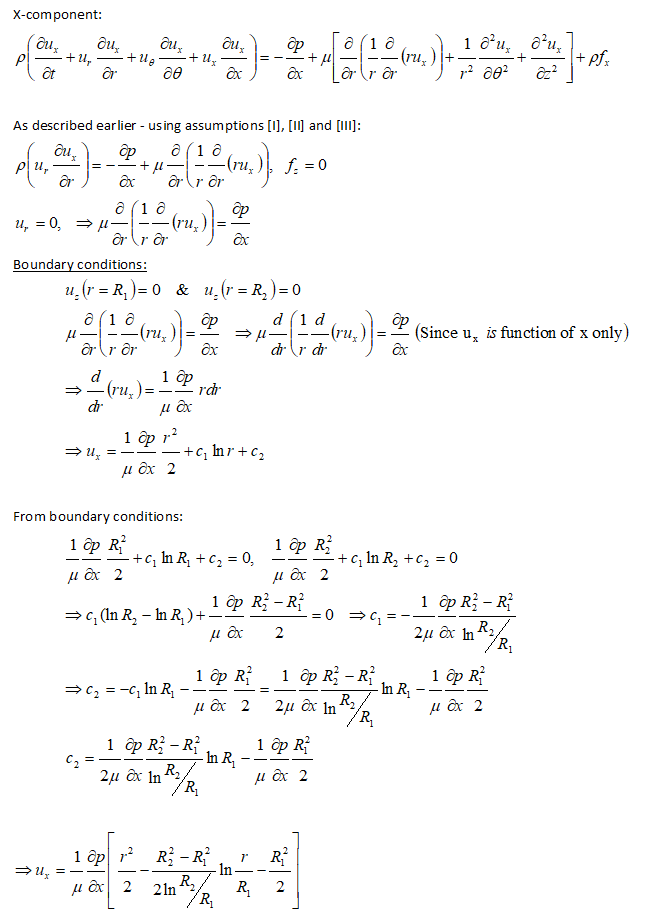#### Flow through Pipe Branches forming Tee or Wye NetworkNote velocities in pipe branches 1-2, 2-3 and 3-4 are not known in advance and hence the loss coefficients KMN cannot be adjusted to same velocity. Note that the junction losses at node 2 can be incorporated either in K12 or in K23 and K24. It is more convenient to club the node loss in K12 as appropriate assignment into K23 and K24 will involve extra calculations.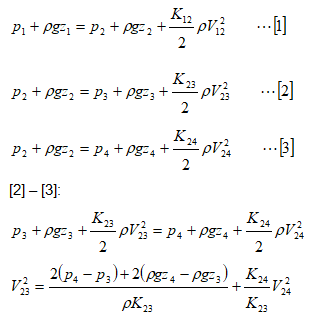Denoting the pressures in terms of head of water column: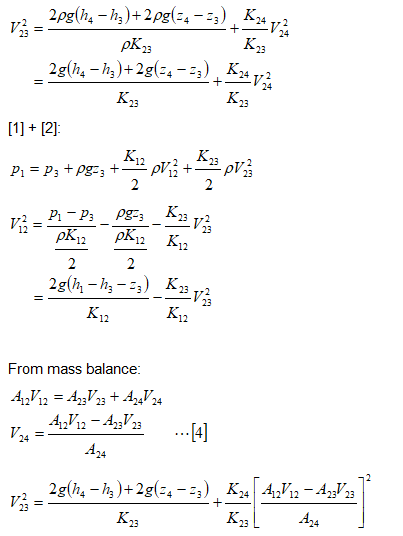Simplifying further: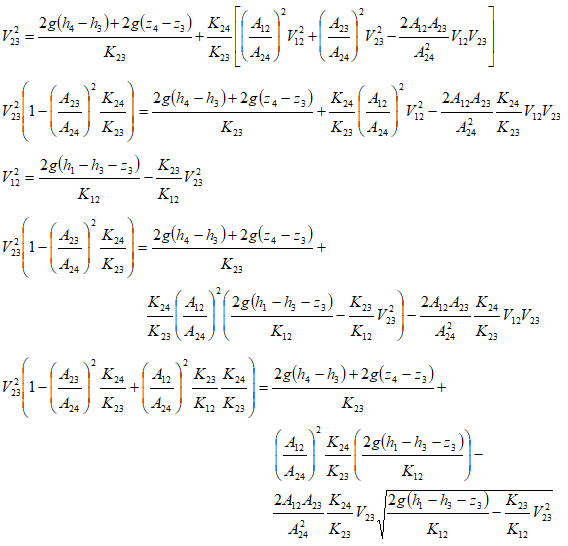This is a non-linear equation in V23 and needs to be solved using trial-and-error or iterative approaches. Microsoft Excel Goal Seek utility can be used to solve this equation as well. A good initial guess would be required.

A more compact approach in terms of fluid resistances can be used as demonstrated below.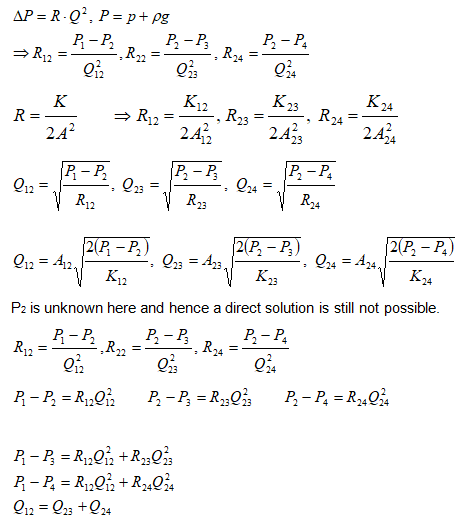Thus, we have 3 equations and 3 unknowns – but they are still non-linear and needs to be solved using iterative method.

#### Force on a curved pipe with varying cross-sectionA reducing bend having diameters D upstream and d downstream, has water flowing through it at the rate of m [kg/s] under a pressure of p1 bar. Neglecting any loss is head for friction calculate, the horizontal and vertical components of force exerted by the water on the bend.

F = m [u2 - u1]

###### Newton's Third Law Applied to Pipe:

This is the force acting on the fluid and only means to apply the force on the fluid is the walls of the pipe. Hence, from Newton’s third law, and equation and opposite force will act on the pipe.

ΔΓX = m * [u2X - u1X] = FΓX. Thus: FΓX = m * [u2 cos(θ) - u1]

ΔΓY = m * [u2Y - u1Y] = FΓY. Hence, FΓY = m * [u2 sin(θ) - 0] = m * u2 sin(θ)
###### Force on fluid element due to pressure at boundaries
FpX = p1A1 - p2A2cos(θ)
FpY = 0 - p2A2sin(θ)

Use Bernoulli’s equation to find p2 in terms of other known parameters: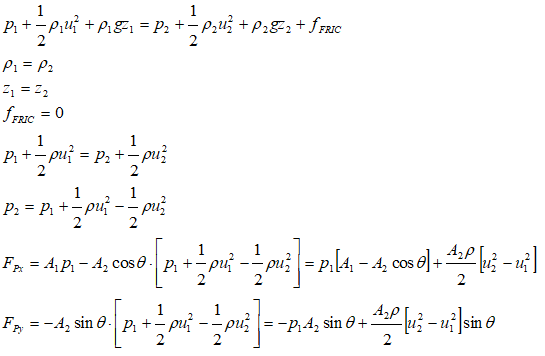From mass balance at inlet and outlet: u2 = u1 * A1 / A2. Thus, we get components of force on fluid elements: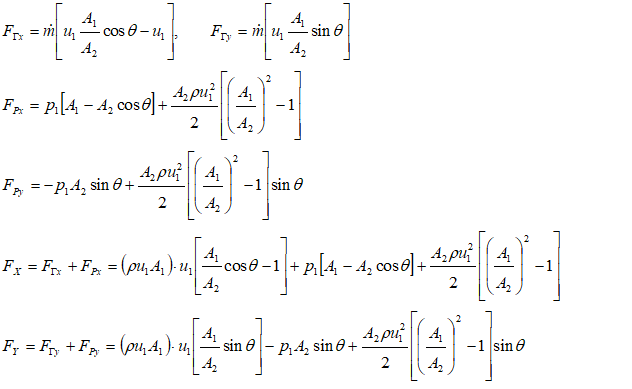As stated earlier, the force on the pipe will be equation in magnitude but opposite in direction. Hence, components of force on pipe are:###### If pipe is straight
θ = 0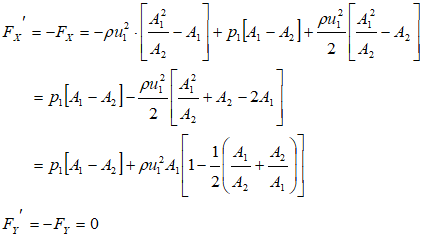#### Equation of State: Soave-Redlick-Kwong Equation

For gases at very high pressure and those in liquid state such as Liquified Petrolium Gas (LPG), the accuracy of ideal gas equation falls sharply. Many improvizations have been made and SRK equation is one such method. The approach is demonstrated using following sample problem:

A gas cylinder with a volume of 5.0 m3 contains 44 kg of carbon dioxide at T = 273 K. estimate the gas pressure in [atm] using the SRK equation of state. Critical properties of CO2 and Pitzer acentric factor are: Tc = 304.2 K, pc = 72.9 atm, and ω = 0.225.

###### General cubic equation of state is given by following equation.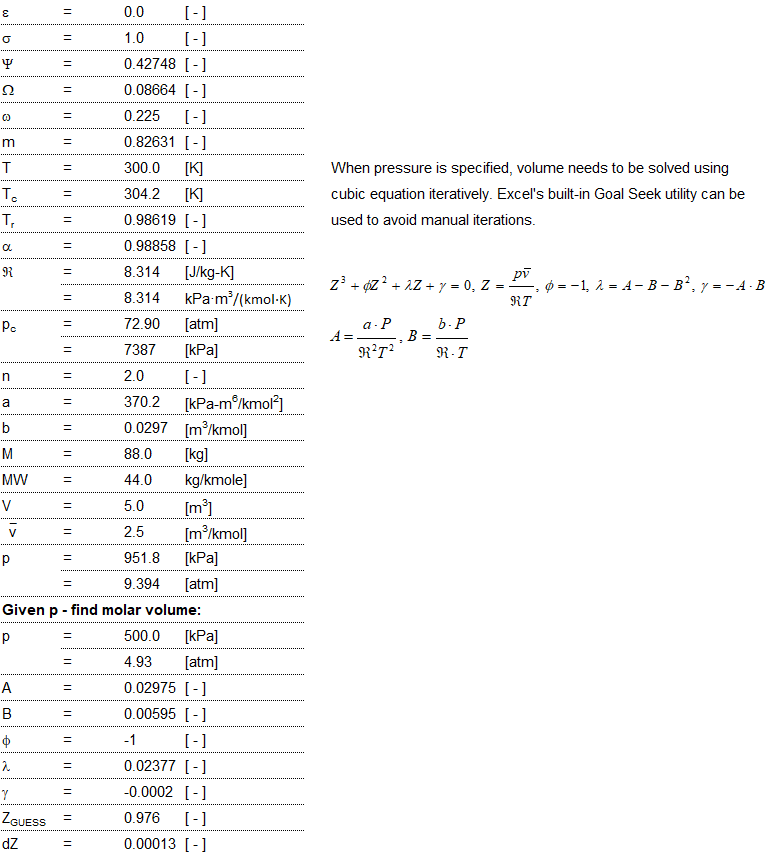###### Alternatively, the equation can be solved using Newton's method as described below.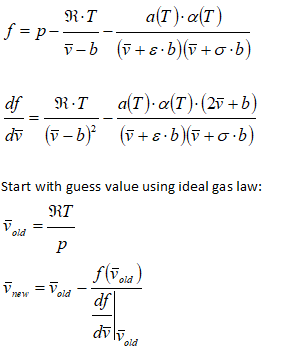Iterate till the difference in vnew and vold falls to desired accuracy.

The content on CFDyna.com is being constantly refined and improvised with on-the-job experience, testing, and training. Examples might be simplified to improve insight into the physics and basic understanding. Linked pages, articles, references, and examples are constantly reviewed to reduce errors, but we cannot warrant full correctness of all content.